# GMAT Math : DSQ: Understanding counting methods

## Example Questions

2 Next →

### Example Question #11 : Counting Methods

A Lunch Deal Meal at the Chinese restaurant where Phyllis likes to eat comprises one appetizer, one entree, and one beverage. How many possible Lunch Deal Meals can Phyllis choose from?

Statement 1: If Phyllis orders either shrimp-based appetizer or one of the three shrimp-based entrees, she will pay extra.

Statement 2: If Phyllis doesn't want shrimp, she has five appetizers and seven entrees to choose from.

Statement 1 ALONE is sufficient to answer the question, but Statement 2 ALONE is NOT sufficient to answer the question.

EITHER statement ALONE is sufficient to answer the question.

BOTH statements TOGETHER are sufficient to answer the question, but NEITHER statement ALONE is sufficient to answer the question.

Statement 2 ALONE is sufficient to answer the question, but Statement 1 ALONE is NOT sufficient to answer the question.

BOTH statements TOGETHER are insufficient to answer the question.

BOTH statements TOGETHER are insufficient to answer the question.

Explanation:

By the multiplication principle, the number of possible Lunch Deal Meals is the product of the number of appetizers, the number of entrees, and the number of beverages. The two statements together give the number of appetizers - two with shrimp and five without for a total of seven - and the number of entrees - three with shrimp and seven without - but not the number of beverages.

### Example Question #11 : Counting Methods

A Lunch Deal Meal at the Japanese restaurant where Mary likes to eat comprises two different entrees and one beverage. How many possible Lunch Deal Meals can Mary choose from?

Statement 1: Mary can choose any of eight different entrees.

Statement 2: Mary can choose as many beverages as entrees.

BOTH statements TOGETHER are insufficient to answer the question.

EITHER statement ALONE is sufficient to answer the question.

BOTH statements TOGETHER are sufficient to answer the question, but NEITHER statement ALONE is sufficient to answer the question.

Statement 2 ALONE is sufficient to answer the question, but Statement 1 ALONE is NOT sufficient to answer the question.

Statement 1 ALONE is sufficient to answer the question, but Statement 2 ALONE is NOT sufficient to answer the question.

BOTH statements TOGETHER are sufficient to answer the question, but NEITHER statement ALONE is sufficient to answer the question.

Explanation:

If there areentrees andbeverages, Mary can select two entrees from theentrees in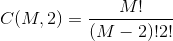ways; by the multiplication principle, she can choose a Lunch Meal Deal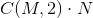ways.

Therefore, the number of entrees and the number of beverages need to be known. Neither statement alone gives both values, but from both statements together, we know that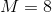and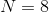, and the number of possible meals can be calculated.

### Example Question #13 : Dsq: Understanding Counting Methods

A Lunch Deal Meal at the Korean restaurant where Vanessa likes to eat comprises one appetizer, one entree, and one beverage. How many possible Lunch Deal Meals can Vanessa choose from?

Statement 1: Vanessa can choose from twice as many entrees as appetizers.

Statement 2: Vanessa can choose from twice as many appetizers as beverages.

Statement 2 ALONE is sufficient to answer the question, but Statement 1 ALONE is NOT sufficient to answer the question.

EITHER statement ALONE is sufficient to answer the question.

BOTH statements TOGETHER are sufficient to answer the question, but NEITHER statement ALONE is sufficient to answer the question.

BOTH statements TOGETHER are insufficient to answer the question.

Statement 1 ALONE is sufficient to answer the question, but Statement 2 ALONE is NOT sufficient to answer the question.

BOTH statements TOGETHER are insufficient to answer the question.

Explanation:

By the multiplication principle, the number of possible Lunch Deal Meals is the product of the number of appetizers, the number of entrees, and the number of beverages. The two statements together only give a relationship among the three, but they do not give any clues as to the actual numbers. Therefore, they give insufficient information.

### Example Question #11 : Counting Methods

A Lunch Deal Meal at the Chinese restaurant where Jing likes to eat comprises one appetizer, one entree, and one beverage. How many possible Lunch Deal Meals can Jing choose from?

Statement 1: The choices include six appetizers, six entrees, and seven beverages.

Statement 2: The Lunch Deal Meal menu is the one shown below: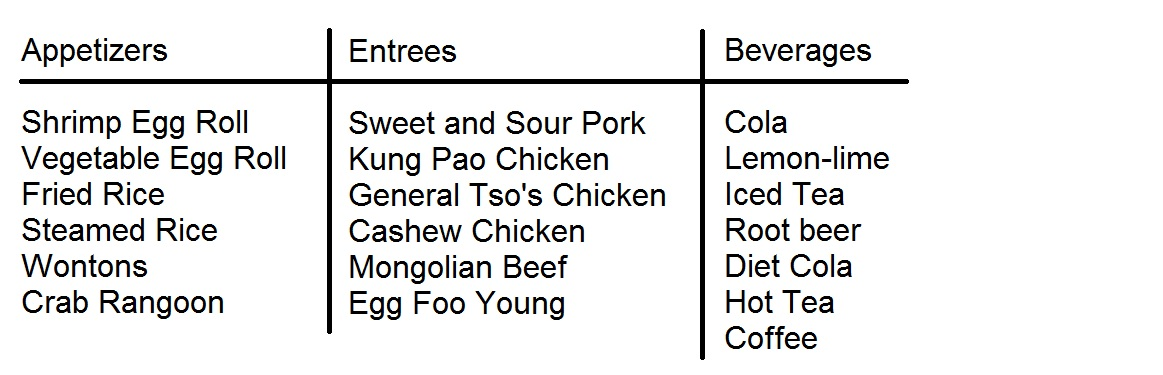EITHER statement ALONE is sufficient to answer the question.

Statement 2 ALONE is sufficient to answer the question, but Statement 1 ALONE is NOT sufficient to answer the question.

BOTH statements TOGETHER are sufficient to answer the question, but NEITHER statement ALONE is sufficient to answer the question.

Statement 1 ALONE is sufficient to answer the question, but Statement 2 ALONE is NOT sufficient to answer the question.

BOTH statements TOGETHER are insufficient to answer the question.

EITHER statement ALONE is sufficient to answer the question.

Explanation:

By the multiplication principle, the number of possible Lunch Deal Meals is the product of the number of appetizers, the number of entrees, and the number of beverages. Each statement alone gives all three numbers - Statement 1 states them explicitly, and Statement 2 gives the actual menu, allowing the number of meals to be calculated as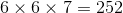.

### Example Question #11 : Counting Methods

A Lunch Deal Meal at the Korean restaurant where Mickey likes to eat comprises one appetizer, one entree, and one beverage. How many possible Lunch Deal Meals can Mickey choose from?

Statement 1: The number of appetizers, the number of entrees, and the number of beverages are the same.

Statement 2: If Mickey orders one of the shrimp dishes, there is a \$1 upcharge; if he orders one of the other six entrees, there is no upcharge.

BOTH statements TOGETHER are insufficient to answer the question.

Statement 2 ALONE is sufficient to answer the question, but Statement 1 ALONE is NOT sufficient to answer the question.

Statement 1 ALONE is sufficient to answer the question, but Statement 2 ALONE is NOT sufficient to answer the question.

EITHER statement ALONE is sufficient to answer the question.

BOTH statements TOGETHER are sufficient to answer the question, but NEITHER statement ALONE is sufficient to answer the question.

BOTH statements TOGETHER are insufficient to answer the question.

Explanation:

By the multiplication principle, the number of possible Lunch Deal Meals is the product of the number of appetizers, the number of entrees, and the number of beverages.

Assume both statements are true. From Statement 1 it can be determined that there are an equal number of choices from each column, but the actual number of choices still needs to be determined. From Statement 2, it can be determined only that there are at least eight entrees - it is given that there are six dishes without shirimp, but the number of dishes with shrimp is not given (only that there must be more than two, since a plural is given). The statements together give insufficient information.

2 Next →

### All GMAT Math Resources